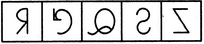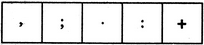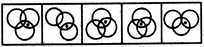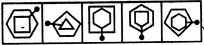# Non Verbal Reasoning - Classification

### Exercise :: Classification - Section 1

In each problem, out of the five figures marked (1), (2), (3), (4) and (5), four are similar in a certain manner. However, one figure is not like the other four. Choose the figure which is different from the rest.

46.

Choose the figure which is different from the rest.(1)     (2)     (3)     (4)     (5)

 A. 1 B. 2 C. 3 D. 4 E. 5

Explanation:

Each one of the figures except fig. (2), is obtained by the lateral inversion of an English alphabet.

47.

Choose the figure which is different from the rest.(1)     (2)     (3)     (4)     (5)

 A. 1 B. 2 C. 3 D. 4 E. 5

Explanation:

All others are punctuation marks.

48.

Choose the figure which is different from the rest.(1)     (2)     (3)     (4)     (5)

 A. 1 B. 2 C. 3 D. 4 E. 5

Explanation:

Only in fig. (4), the dot appears in the region common to all the three circles.

49.

Choose the figure which is different from the rest.(1)     (2)     (3)     (4)     (5)

 A. 1 B. 2 C. 3 D. 4 E. 5